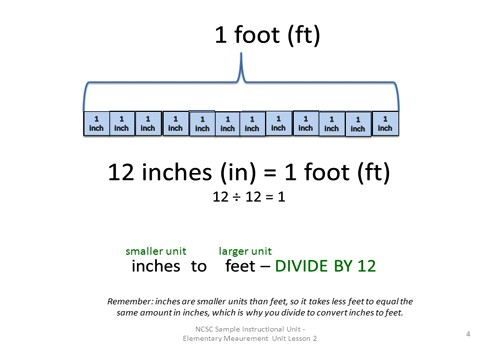Feet and inches are US customary system units, so let’s look at information that is as close to official as it can be, material from the National Institute of Standards and Technology, rather than depending on opinions of various people, who might or might not be well-informed on the topic.

Convert feet and inches to inches by multiplying feet by 12 and adding the number of inches:

• Perform the required division to determine the number of inches.
• Convert the inches to feet and inches by dividing by 12.
• The quotient is the number of feet and the remainder is the number of inches.Example: Divide 10 feet 0 inches by 4

Convert 10 feet to inches by multiplying 12 times 10:

12 * 10 = 120 inches

Add the number of extra inches:

120 + 0 = 120 inches

Perform the required division:

120 ÷ 4 = 30 inches

Convert to feet and inches by dividing by 12:

30 ÷ 12 = 2 R 6

The quotient (2) is the number of feet and the remainder (6) is the number of inches.

The international standard symbol for a foot is “ft” (see ISO 31-1, Annex A). In some cases, the foot is denoted by a prime, which is often marked by an apostrophe, and the inch by a double prime; for example, 2 feet 4 inches is sometimes denoted as 2′−4″, 2′ 4″ or 2′4″.

12 Inches equal 1 Foot

3 Feet equal 1 Yard

220 Yards equal 1 Furlong

5 Furlongs equal 1 Kilometer

1.6 Kilometers equal 1 Mile.

http://www.aaamath.com/div_ftin.htm